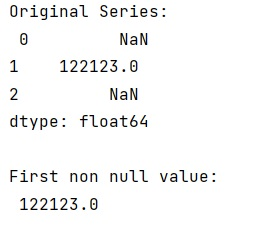# Find first non-null value in column

Given a pandas dataframe, we have to find the first non-null value in column.
Submitted by Pranit Sharma, on November 09, 2022

Pandas is a special tool that allows us to perform complex manipulations of data effectively and efficiently. Inside pandas, we mostly deal with a dataset in the form of DataFrame. DataFrames are 2-dimensional data structures in pandas. DataFrames consist of rows, columns, and data.

## Problem statement

We are given a series that has either NULL or some non-null value. We need to find the 1st row where the value is not null so that we can use this data. If the values are not null all the values are the same data type in that series.

## Finding the first non-null value in column

For this purpose, we will first create a series of integer values along with some null values. We are going to use first_valid_index() method which will return that value location that has a non-null value.

Also, by applying this method inside loc[] property, we will find that particular value.

Let us understand with the help of an example,

## Python program to find the first non-null value in column

```# Importing pandas package
import pandas as pd

# Importing numpy package
import numpy as np

# Creating a series
s = pd.Series([np.nan,122123,np.nan])

# Display series
print('Original Series:\n',s,'\n')

# Finding first non null value
res = s.first_valid_index()

# Finding location
res_loc = s.loc[s.first_valid_index()]

# Display result
print('First non null value:\n',res_loc,'\n')
```

## Output